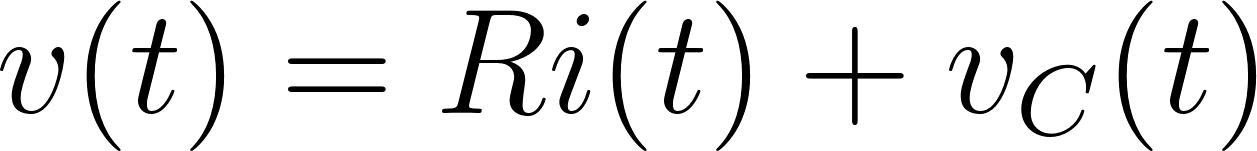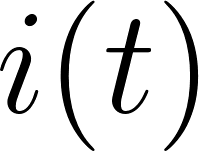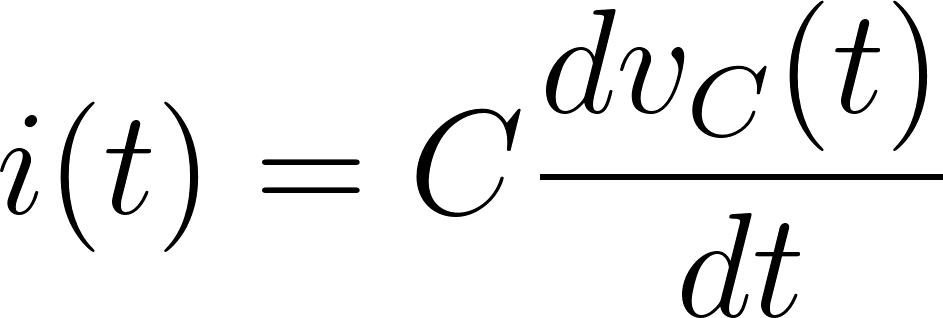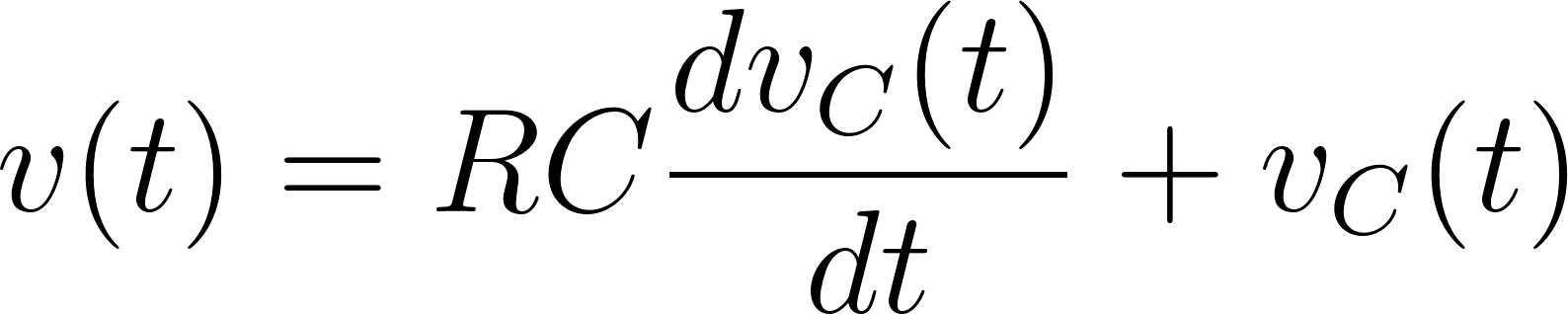Introduction To Electric Circuits - 9 Edition - Chapter 7.2 - Problem P7.2-18
Register Now

Join StudySoup

Get Full Access to Introduction To Electric Circuits - 9 Edition - Chapter 7.2 - Problem P7.2-189781118477502

# The input to the circuit shown in Figure P 7.2-18 is the current i t 3e25t A for t > 0

Introduction to Electric Circuits | 9th Edition

Problem P7.2-18

The input to the circuit shown in Figure P 7.2-18 is the current i t 3e25t A for t > 0 The output is the voltage v t 9:6e25t 0:4 V for t > 0 The initial capacitor voltage is vC 0 2 V. Determine the values of the capacitance C and resistance R. C + v(t) _ vC + (t) _ i(t) R Figure P 7.2-18 P

Accepted Solution
Step-by-Step Solution:

Step 1 of 4

Let's start by writing a KVL equation for this loop.(equation 1)

The currentflows through the capacitor, we know that.

So,###### Chapter 7.2, Problem P7.2-18 is Solved

Step 2 of 4

Step 3 of 4

Unlock Textbook Solution